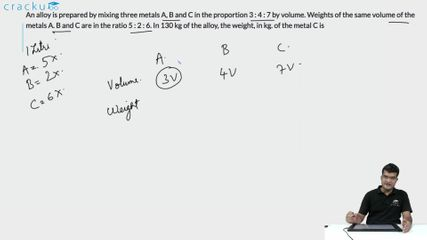Question 15

# An alloy is prepared by mixing three metals A, B and C in the proportion 3 : 4 : 7 by volume. Weights of the same volume of the metals A. B and C are in the ratio 5 : 2 : 6. In 130 kg of the alloy, the weight, in kg. of the metal C is

Solution

Let the volume of Metals A,B,C we 3x, 4x, 7x

Ratio weights of given volume be 5y,2y,6y

.'. 15xy+8xy+42xy=130 => 65xy=130 => xy=2.

.'.`The weight, in kg. of the metal C is 42xy=84.

### View Video Solution